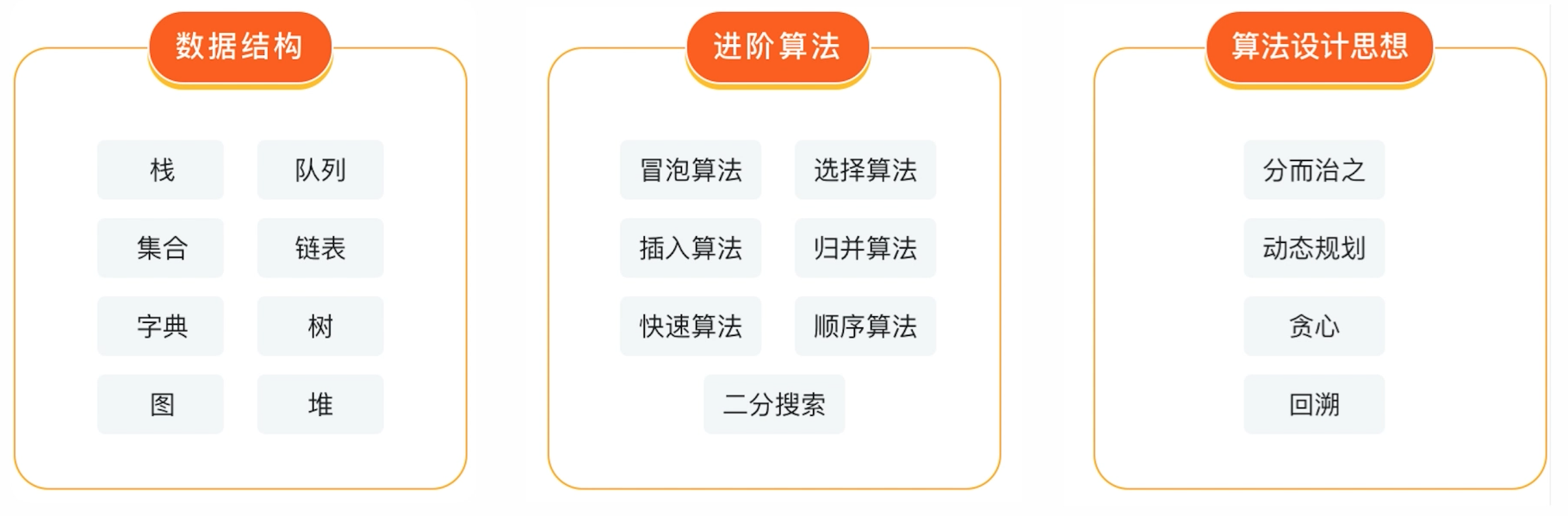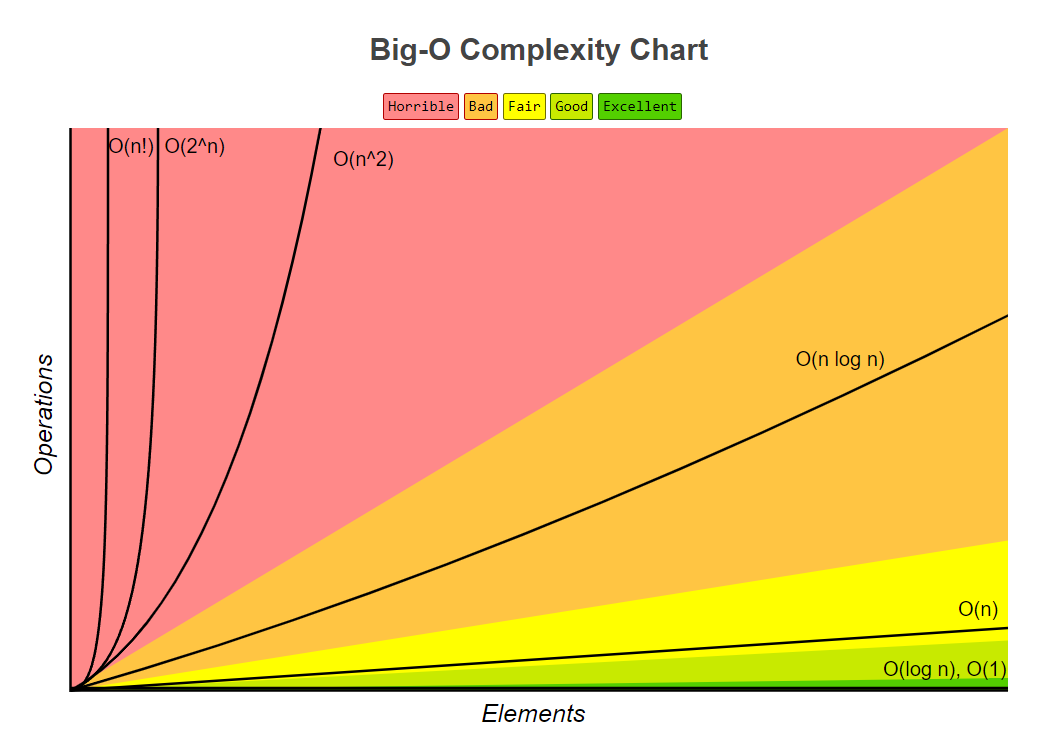📢 大家好，我是小丞同学，一名大二的前端爱好者

📢 这篇文章是数据结构与算法专栏的第一篇博文

📢 非常感谢你的阅读，不对的地方欢迎指正 🙏

📢 愿你忠于自己，热爱生活

• 算法基础
• 计算时间复杂度
• 计算空间复杂度
• 数据结构和算法的学习指南

❗❗❗ 文末有惊喜噢~

## 专栏简介 ​（图片来源于慕课网截图）

## 引言 ​

• 为了计算出最优解## 一. 大 O 表示法 ​

O(1)常数
O(log N)对数
O(N)线性
O(N log N)对数多项式
O(N^2)二次
O(2^N)指数
O(N!)阶乘

js
``````function test(num){
return ++num;
}``````

## 二. 时间复杂度 ​

`O` 表示法表明的是该段代码执行时间随数据规模增大的变化趋势，它的特点是

• 只关注量级最大的时间复杂度

### 1. O(1) ​

js
``````let i = 0;
i += 1;
// 每次执行代码只执行一次 O(1)``````

### 2. O(n) ​

js
``````for (let j = 0; j < n; j++) {
console.log(j);
}``````

js
``````let i = 0;
i += 1;
for (let j = 0; j < navigator; j++) {
console.log(j);
}``````

• 因此它的时间复杂度同样是 `O(n)`

### 3. O(log(n)) ​

js
``````while (i < n) {
console.log(i);
i *= 2;
}``````

### 4. O(n^2) ​

js
``````for (let i = 0; i < n; i++) {
for (let j = 0; j < n; j++) {
console.log(i);
}
}``````## 三、空间复杂度 ​

### 1. O(1) ​

js
``let i = 0;``

### 2. O(n) ​

js
``````let arr = []
for(let i = 0;i < n;i++) {
arr.push(i)
}``````

### 3. O(n^2) ​

js
``````int arr = [][]
// 遍历赋值``````

## 📌 总结 ​

1. 复杂度计算按最高阶来计算
2. 时间、空间复杂度描述的都是随数据规模的变化趋势
3. 时间复杂度的重点在于循环嵌套
4. 空间复杂度关注于内存

## 🐣 博主有话说 ​

### 2. 说说为什么要学数据结构和算法吧？ ​

1. 第一点：如文章开头所言，我想要写出最优的代码，这点是个人观念的原因，在学习了 `ES6+` 语法之后，以前很多的代码都显得冗余复杂了，`map``set` 就是最好的例子

2. 第二点：提高代码运行效率，这一点不仅仅体现在刷算法题上，更体现在实际场景中，我们可能会因为我们的算法问题导致了时间复杂度过高，导致发送无用请求，导致前台页面等待时间过长等问题，这些都是我们前端需要懂得优化的

3. 第三点：为了面试，这就很真实了，在网上看了很多的面经，算法都是必要的一环，所以学习好数据结构和算法也能为自己以后面试提前做好准备

### 3. 学习资料分享 ​

💌 最后，可能在很多地方讲诉的不够清晰，请见谅

💌 如果文章有什么错误的地方，或者有什么疑问，欢迎留言，也欢迎私信交流

Last updated: